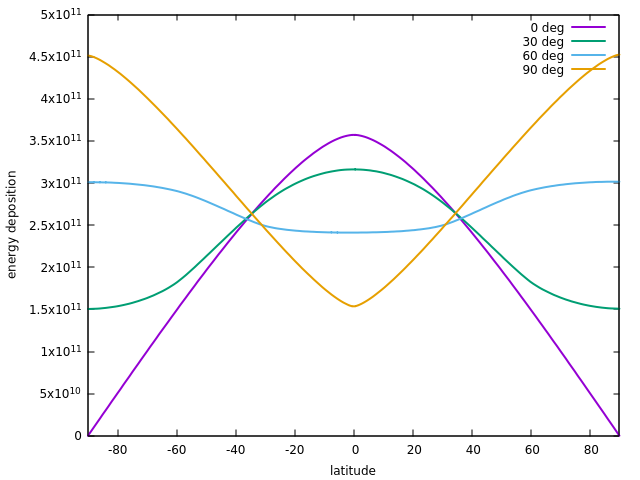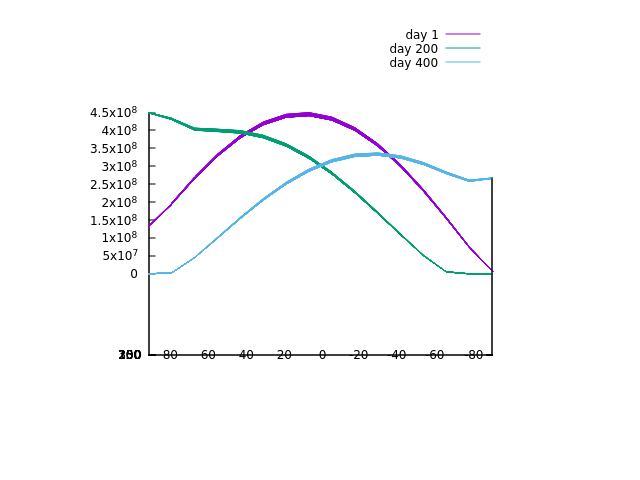With the energy output and the orbit of the planet known, we can next ask the question of much energy reaches each part of the planetary surface. The answer to that question depends on multiple factors. First, if the orbit is eccentric, there is more energy received when the planet is close to the star. Second, due to the spherical shape of a planet, surface elements that receive light under a shallow angle. On Earth, this gives rise to climate zones, the polar regions are colder than the tropics. Third, the axial tilt with respect to the orbital plane gives rise to seasons - and if the axial tilt is very extreme (such as for Uranus), the energy deposition is actually highest at the poles. Finally, part of the energy may simply not be received at all but reflected back into space - the fraction at which that happens is the albedo. High albedo surfaces such as fresh snow may reflect up to 90% of the energy back into space.

Note that going from 'absorbed energy' to 'temperature' is far from trivial - generally the temperature is not just the radiative equilibrium temperature for which incoming radiation equals outgoing radiation, but for a day/night cycle there is thermal inertia to consider: Materials do not immediately cool to absolute zero because they no longer receive radiation. Then there is the role of the atmosphere which through the Greenhouse effect may absorb outgoing but not incoming radiation, and then there is energy transport through water masses and atmosphere which also have a huge influence on a temperature profile. For these reasons, we will first cover irradiation and discuss temperatures later.

The simple case - fast rotation

If a planet rotates reasonably fast compared with its orbital motion, i.e. if the day is much shorter than the year, then to very good approximation all longitudes of the planet receive radiation the same way and mean irradiation becomes a function of latitude only. For the question of climate zones, we're chielfy interested inaveraging the received energy over the time of one orbit.

Note that e.g. for a tidally locked planet where the sidereal rotation is as long as the orbital period, only one hemisphere ever faces towards the star and receives radiation. In this situation irradiation is evidently not independent of longitude, and we will cover this situation later.

The irradiation function dependent on latitude can be computed via a plot block as follows:

 plot var_x lat var_y year_avg_irradiation xmin -90 xmax 90 npoints 180 file irradiation.dat

This plots the received energy as a function of latitude from -90 to +90 degrees, summed over a whole orbit.

Doing this e.g. for the Mars orbit and varying the axial tilt from 0 to 90 degrees reveals that while for small tilt values most energy is deposited in the tropics, this changes for high axial tilts - somewhere beyond 60 degrees, most energy reaches the poles.Irradiation as a function of latitude for different axial tilt

Seasons

The whole energy deposition over the course of an orbit is not necessarily too useful. However, for a more differential picture of the situation, a more detailed simulation mode has to be used. For this, the surface of the planet gets subdivided into angular regions in a grid of latitude and longitude.

We then would like to advance the simulation in orbit to a certain position, then record the energy deposition as it happens for a period of 30 days at that position. Finally, the recorded energy deposition should be written out as a surface plot. This can be accomplished with the following lines (for illustration, the axis tilt of Mars is here changed to 30 degrees).

 planet name Mars mass 0.107 radius 0.532 semimajor_au 1.523 eccentricity 0.0934 axis_tilt 30 sid_rot_period_d 1.0259 mean_albedo 0.25 elements_lat 16 elements_lon 24 orbit evolve_d 100 timestep 1000 evolution evolve_d 30 timestep 1000.0 plot_surface file irr_surf_100.dat var_z total_irradiation

The keywords elements_lat and elements_lon initialize the subdivision of the planetary surface at the chosen resolution.

The keyword orbit runs the orbital solver only, evolve_d specifies the number of days for which the orbital solver runs, timestep specifies the timestep for the orbital solver in seconds.

In contrast, the keyword evolution does a full evolution (orbital motion and radiation/thermal environment) - which is executed after the orbital solver has done its job.

Finally, plot_surface writes the recorded data to a specified file. Here setting var_z to total_irradiation selects the total deposited energy during the evolution phase as the variable of interest.

Doing the simulation for 1, 200 and 400 days of orbital motion before the 30 day full evolution yields, when the 2d data is projected into a latitude plot, the following pattern:Irradiation as a function of latitude 30 deg axial tilt after different time periods

One can see how, dependent on the position along the orbital track, the tropical zone, the northern or the southern hemisphere receives more light.

In this way, the developing patterns of seasons along the orbit of the planet can be studied.